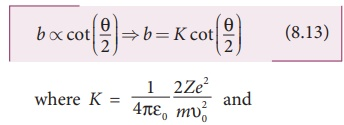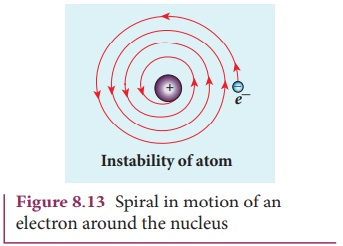Home | | Physics 12th Std | Rutherford’s model

# Rutherford’s model

The alpha particles coming through the fine hole of lead box pass through another fine hole made on the lead screen.

ATOM MODELS

Rutherford’s model

In 1911, Geiger and Marsden did a remarkable experiment based on the advice of their teacher Rutherford, which is known as scattering of alpha particles by gold foil.

The experimental arrangement is shown in Figure 8.9. A source of alpha particles (radioactive material, example polonium) is kept inside a thick lead box with a fine hole as seen in Figure 8.9.The alpha particles coming through the fine hole of lead box pass through another fine hole made on the lead screen. These particles are now allowed to fall on a thin gold foil and it is observed that the alpha particles passing through gold foil are scattered through different angles. A movable screen (from 0° to 180°) which is made up of zinc sulphide (ZnS) is kept on the other side of the gold foil to collect the alpha particles. Whenever alpha particles strike the screen, a flash of light is observed which can be seen through a microscope.Rutherford proposed an atom model based on the results of alpha scattering experiment. In this experiment, alpha particles (positively charged particles) are allowed to fall on the atoms of a metallic gold foil. The results of this experiment are given below and are shown in Figure 8.10, Rutherford expected the nuclear model to be as seen in Figure 8.10 (a) but the experiment showed the model as in Figure 8.10 (b).

(a) Most of the alpha particles are un- deflected through the gold foil and went straight.

(b) Some of the alpha particles are deflected through a small angle.

(c) A few alpha particles (one in thousand) are deflected through the angle more than 90°

(d) Very few alpha particles returned back (back scattered) –that is, deflected back by 180°

In Figure 8.10 (c), the dotted points are the alpha scattering experiment data points obtained by Geiger and Marsden and the solid curve is the prediction from Rutherford’s nuclear model. It is observed that the Rutherford’s nuclear model is in good agreement with the experimental data.

### Conclusion made by Rutherford based on the above observation

From the experimental observations, Rutherford proposed that an atom has a lot of empty space and contains a tiny matter known as nucleus whose size is of the order of 10-14m. The nucleus is positively charged and most of the mass of the atom is concentrated in nucleus. The nucleus is surrounded by negatively charged electrons. Since static charge distribution cannot be in a stable equilibrium, he suggested that the electrons are not at rest and they revolve around the nucleus in circular orbits like planets revolving around the sun.

### (a) Distance of closest approachWhen an alpha particle moves straight towards the nucleus, it reaches a point where it comes to rest momentarily and returns back as shown in Figure 8.11. The minimum distance between the centre of the nucleus and the alpha particle just before it gets reflected back through 180° is defined as the distance of closest approach r0 (also known as contact distance). At this distance, all the kinetic energy of the alpha particle will be converted into electrostatic potential energy (Refer unit 1, volume 1 of +2 physics text book).where Ek is the kinetic energy of the alpha particle.This is used to estimate the size of the nucleus but size of the nucleus is always lesser than the distance of closest approach. Further, Rutherford calculated the radius of the nucleus for different nuclei and found that it ranges from 10–14 m to 10–15 m.

### (b) Impact parameterThe impact parameter (b) (see Figure 8.12) is defined as the perpendicular distance between the centre of the gold nucleus and the direction of velocity vector of alpha particle when it is at a large distance. The relation between impact parameter and scattering angle can be shown asand θ is called scattering angle. Equation (8.13) impliesthat when impact parameter increases, the scattering angle decreases. Smaller the impact parameter, larger will be the deflection of alpha particles.

### Drawbacks of Rutherford model

Rutherford atom model helps in the calculation of the diameter of the nucleus and also the size of the atom but has the following limitations:

(a) This model fails to explain the distribution of electrons around the nucleus and also the stability of the atom.According to classical electrodynamics, any accelerated charge emits electromagnetic radiations. Due to emission of radiations, it loses its energy. Hence, it can no longer sustain the circular motion. The radius of the orbit, therefore, becomes smaller and smaller (undergoes spiral motion) as shown in Figure 8.13 and finally the electron should fall into the nucleus and the atoms should disintegrate. But this does not happen.

Hence, Rutherford model could not account for the stability of atoms.

(b) According to this model, emission of radiation must be continuous and must give continuous emission spectrum but experimentally we observe only line (discrete) emission spectrum for atoms.

Tags : Atom Models | Physics , 12th Physics : UNIT 9 : Atomic and Nuclear Physics
Study Material, Lecturing Notes, Assignment, Reference, Wiki description explanation, brief detail
12th Physics : UNIT 9 : Atomic and Nuclear Physics : Rutherford’s model | Atom Models | Physics